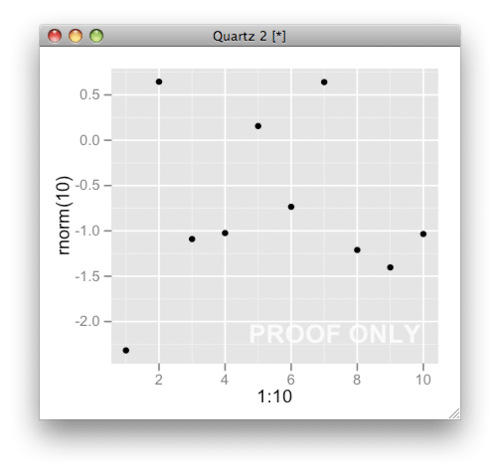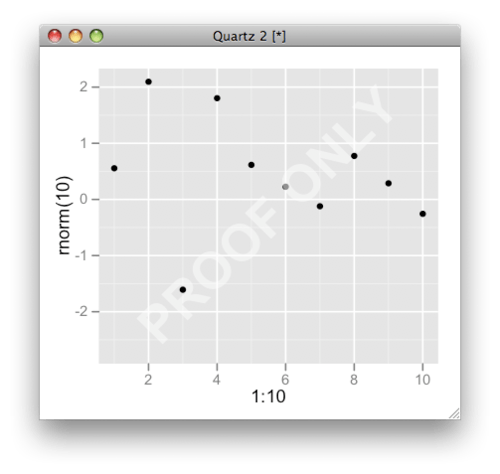Want to share your content on R-bloggers? click here if you have a blog, or here if you don't.

A question was raised today on the mailing list: Is there an easy way to add a watermark to a ggplot?

There are several options, depending on the type of watermark and the required level of control over the output,

• add a text label using `annotate` (the original idea of the poster)

• add a custom grob (graphical object from the Grid package), using `annotation_custom`

In either case, the placement of a watermark at an absolute location on the plot is greatly facilitated if you use +/- Inf values, which correspond to the extreme edges of the plot panel.

Here is an example with `annotate`

``` library(ggplot2)
library(grid)

qplot(1:10, rnorm(10)) +
annotate("text", x = Inf, y = -Inf, label = "PROOF ONLY",
hjust=1.1, vjust=-1.1, col="white", cex=6,
fontface = "bold", alpha = 0.8)
```

where the label is placed at the bottom-right, and the justification is adjusted to make sure the label stays in the panel area.Below is a fancier example with a custom grob, which we define such that its width spans the full plot panel, even after resizing the interactive plot window,

``` watermarkGrob <- function(lab = "PROOF ONLY"){
grob(lab=lab, cl="watermark")
}

## custom draw method to
## calculate expansion factor on-the-fly
drawDetails.watermark <- function(x, rot = 45, ...){
cex <- convertUnit(unit(1,"npc"), "mm", val=TRUE) /
convertUnit(unit(1,"grobwidth", textGrob(x\$val)), "mm",val=TRUE)

grid.text(x\$lab,  rot=rot, gp=gpar(cex = cex, col="white",
fontface = "bold", alpha = 0.5))

}

qplot(1:10, rnorm(10)) +
annotation_custom(xmin=-Inf, ymin=-Inf, xmax=Inf, ymax=Inf, watermarkGrob())
```You can of course replace this grob with a more complex one, e.g a table of labels to tile the panel with multiple repetitions of the watermark, or an external graphic (consider the `annotation_raster` function), etc. As an example, the following function uses `rpatternGrob` from the gridExtra package to tile multiple copies of the R logo, imported as a raster image,

``` library(png)
library(gridExtra)
## import logo as raster image
m <- readPNG(system.file("img", "Rlogo.png", package="png"), FALSE)
w <- matrix(rgb(m[,,1],m[,,2],m[,,3], m[,,4] * 0.2), # adjust alpha
nrow=dim(m))

qplot(1:10, rnorm(10), geom = "blank") +
annotation_custom(xmin=-Inf, ymin=-Inf, xmax=Inf, ymax=Inf,
rpatternGrob(motif=w, motif.width = unit(1, "cm"))) +
geom_point()
```This time we made sure that the logo was the first layer plotted, so that it doesn’t obfuscate the data but stays in the background.